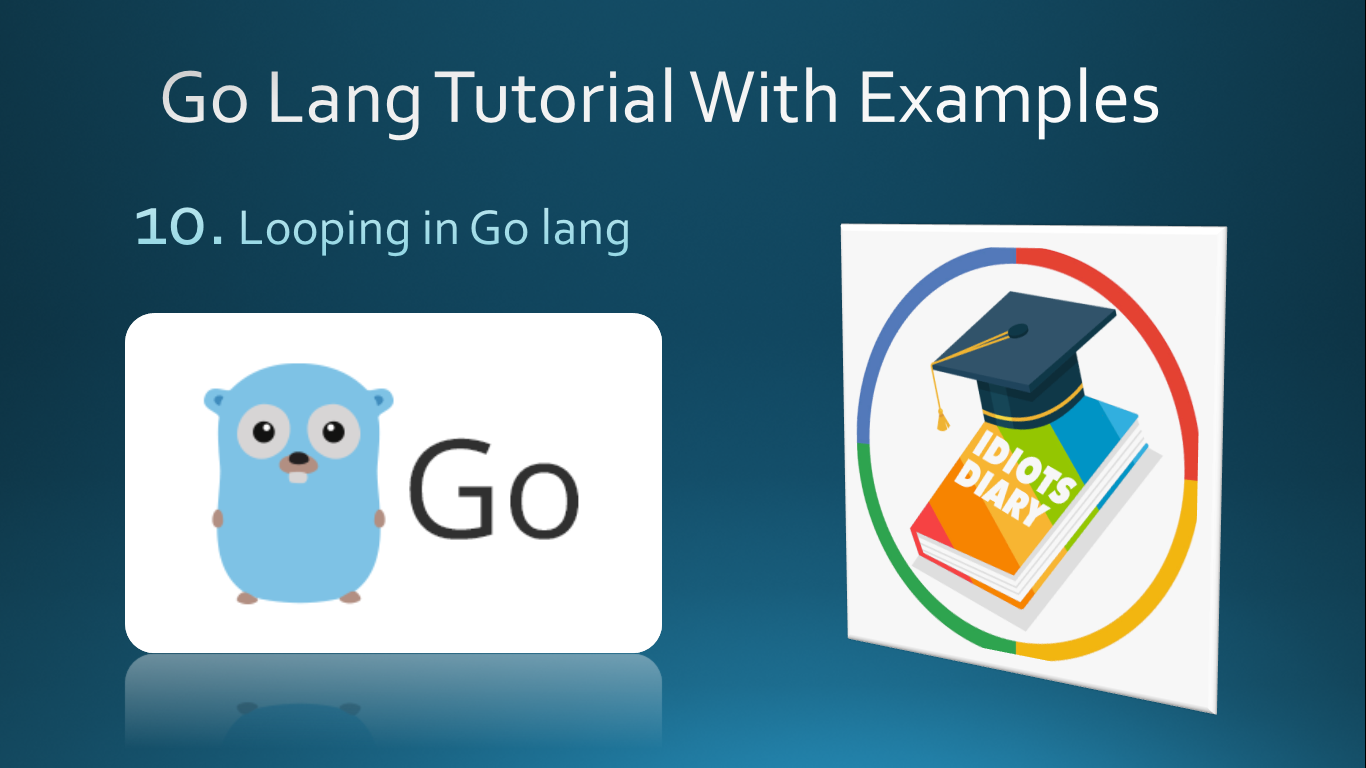## Posts

Showing posts with the label Go lang by example

### Looping in go langLoop is a structure with the block of statements can be repeated for the finite number of times.
Till Now we have learned the programs that execute sequentially, Now we are going to learn control structures which change the normal flow of the program and helps you to create more meaningful and compact program structure.

To understand more, suppose we want to print from 1 to 10 number counting, each number should be printed in the new line.
Program to print numbers from 1 to 10 package main
import "fmt"
func main( ) {
fmt.Println(1)
fmt.Println(2)
fmt.Println(3)
fmt.Println(4)
fmt.Println(5)
fmt.Println(6)
fmt.Println(7)
fmt.Println(8)
fmt.Println(9)
fmt.Println(10)
} Another compact way to create this program use loop. The following example creates this program using the loop. Program package main
import "fmt"
func main() {
i := 1 //initialization
for i <= 10 { //condition
fmt.Println(i) //statement
i = i + 1 //updates
}
} Output 1
2
3
4
5
6
7
8
9
10With the above program sn…

### Convert Temperature from Fahrenheit to centigrade

The formula to convert temperature from Fahrenheit to Centigrade is as below...C = ( F - 32 ) * 5 / 9
where C and F are temperature in Centigrade and Fahrenheit in respectively. package main
import "fmt"
func main() {
var f float32
fmt.Print("Enter the temperature in fahrenheit: ")
fmt.Scan(&f)
c := ( f - 32 ) * 5 / 9
}

### Constant in Go langConstant in go langConstants are basically variable which value can't change once created.Constants are created in the same way as variables, the only difference is that to create a variable use var keyword and to create constant use const keyword. Constant Example-1 package main
import "fmt"
func main() {
const playerName string = "Rahul"
fmt.Println(playerName)
//playerName = "Micky" //compile time error, cannot assign to playerName.
} Constant Example-2 package main
import "fmt"
func main() {
const METER_PER_FEET = 0.3048
var feet,meter float32
fmt.Print("Enter value in feet...")
fmt.Scan(&feet)
meter = feet * METER_PER_FEET
fmt.Printf("Meter %.2f",meter)
}  Note:In the above example, METER_PER_FEET is a constant.It is suggested that constant name should be written in uppercase, each word separated by an underscore so can be easily identified.

### Simple interest in go lang

package main
import "fmt"
func main() {
var p int
var r float32
var t int
fmt.Print("Enter principal, rate and time: ")
fmt.Scan(&p,&r,&t)
si := float32(p) * float32(t) * r / 100
fmt.Printf("Simple interest: %.2f",si)
} Explanation  Most of the things of the above program are already discussed. bur some of the important statement is discussed here. si := float32(p) * float32(t) * r / 100
The above program statement performs a simple calculation p * t * r / 100 and stores the result in variable si. In go lang in a single expression multiple types are not allowed, so we need to convert p and t into float32 type and expression p * t * r becomes a float32 type expression.To typecast a variable we use the following syntax type(var)
In the above program snippet to print the result fmt.Printf("Simple interest : %.2f",si).Printf() is a function used to print the formatted output.%.2f prints a floating point value with only 2 decimal places.

### Identify Variable type and values in go lang

package main
import "fmt"
func main() {
var name string = "Mahesh"
age := 25
price := 300.40
fmt.Printf("%T %v\n",name,name)
fmt.Printf("%T %v\n",age,age)
fmt.Printf("%T %v\n",price,price)
} Output string Mahesh
int 25
float64 300.4
ExplanationIn the above program snippet, we use Printf() functionof fmt package to print the formatted output.Withing the Printf( ) %T and %v is called format specifier which represents the output format.%T represents the Type, which prints the variable type%v represents the variable's value.age := 25, creates a variable age with the short variable declaration and assign the value 25. In this case, the variable automatically receives the type and here age is the type of int.price:=300.40, In this statement price, is created of type float64 because float64 is the default type of floating values in go lang. To create a float32 type variable you need to create a variable as given below.\n is called escape seque…

### Sum of two numbers in go lang

package main
import "fmt"
func main() {
var a,b int
a = 5
b = 10
sum := a + b
fmt.Println("Total = ",sum)
} Explanationvar a,b int: declare two variable a and b. Learn here how to create variablea = 5 and b = 10 assign 5,10 to a,b respectivelysum:= a + b:  adds a and b and store the result in sum as well as created sum variable with the short variable declaration (:=). fmt.Println("Total = ",sum): prints the result as Total = 15 ImprovementsAbove program always returns fixed result Total = 15, because a and b are initialized with fixed value 5, 10 respectively.So this program can be improved by using user input.As well as above program variable declaration and initialization can be done in a single statement as shown here var a,b int = 5 , 10
OR
var a,b = 5 , 10 Sum of two numbers with user input package main
import "fmt"
func main() {
var a,b int
fmt.Print("Enter any two numbers: ")
fmt.Scan(&a,&b)
sum := a + b
fmt.Println("Total …

### Program to print Hello world in go lang

package main
import "fmt"
func main() {
fmt.Println("Hello Go Lang")
} Output  Hello Go lang ExplanationIf you are a beginner an don't know how to install go lang and run the program, you can learn installation with the installation of go lang.To read the complete explanation of the above program visit, How to print Hello world in go lang.